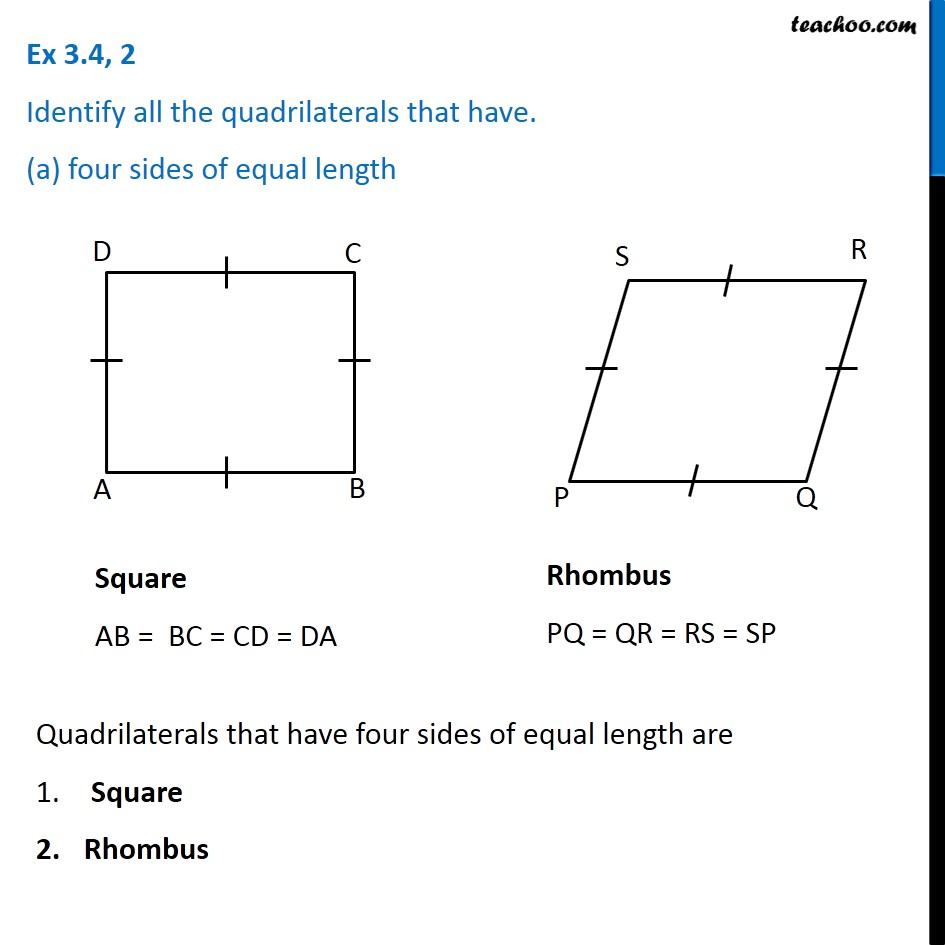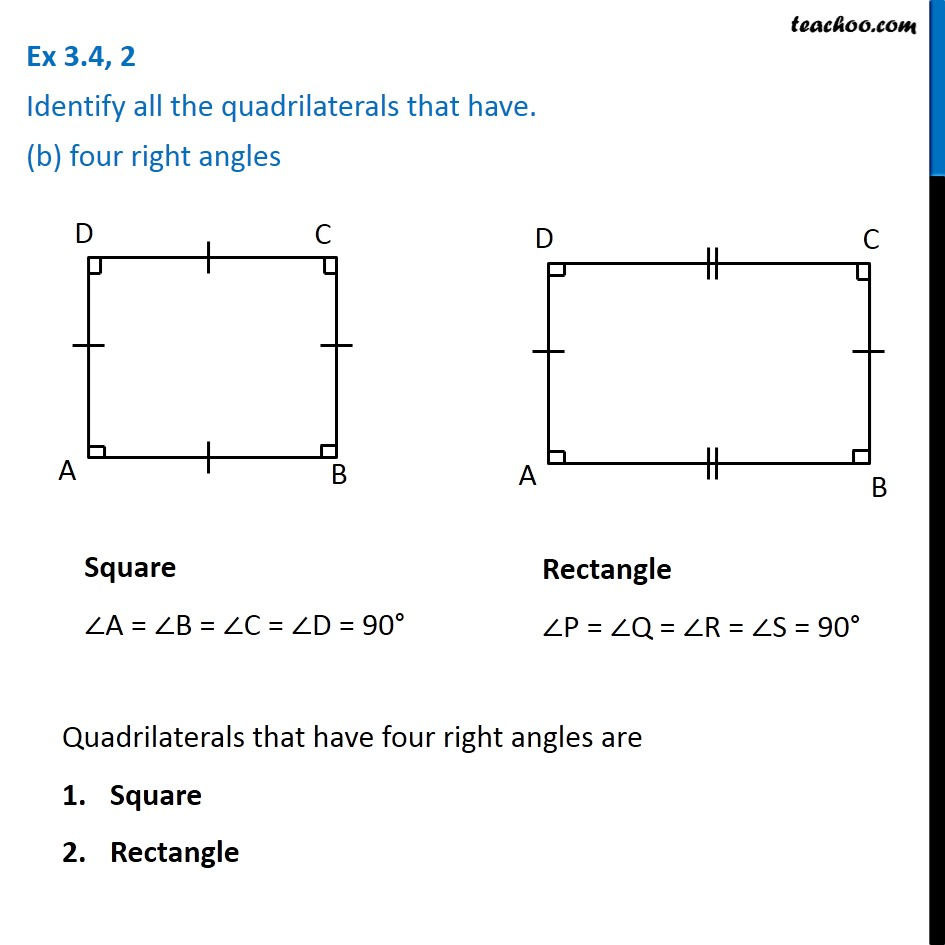Ex 3.4

Chapter 3 Class 8 Understanding Quadrilaterals
Serial order wiseLearn in your speed, with individual attention - Teachoo Maths 1-on-1 Class

### Transcript

Ex 3.4, 2 Identify all the quadrilaterals that have. (a) four sides of equal length Square AB = BC = CD = DA Rhombus PQ = QR = RS = SP Quadrilaterals that have four sides of equal length are Square Rhombus Ex 3.4, 2 Identify all the quadrilaterals that have. (b) four right angles Square ∠A = ∠B = ∠C = ∠D = 90° Rectangle ∠P = ∠Q = ∠R = ∠S = 90° Quadrilaterals that have four right angles are Square Rectangle